# Surface Areas and Volumes Class 10 Notes

According to the CBSE Syllabus 2023-24, this chapter has been renumbered as Chapter 12.

## CBSE Class 10 Maths Surface Area and Volumes Notes:-

The concept of surface area and volume for Class 10 is provided here. In this article, we are going to discuss the surface area and volume for different solid shapes such as the cube, cuboid, cone, cylinder, and so on. The surface area can be generally classified into Lateral Surface Area (LSA), Total Surface Area (TSA), and Curved Surface Area (CSA). Here, let us discuss the surface area formulas and volume formulas for different three-dimensional shapes in detail. In this chapter, the combination of different solid shapes can be studied. Also, the procedure to find the volume and its surface area in detail.

To get the solutions for class 10 Maths surface areas and volumes, click on the below link.

## Video Lesson on Surface Areas and Volumes Class 10## Surface Area and Volume of Cuboid

A cuboid is a region covered by its six rectangular faces. The surface area of a cuboid is equal to the sum of the areas of its six rectangular faces.

### Surface Area of the Cuboid

Consider a cuboid whose dimensions are × × h, respectively.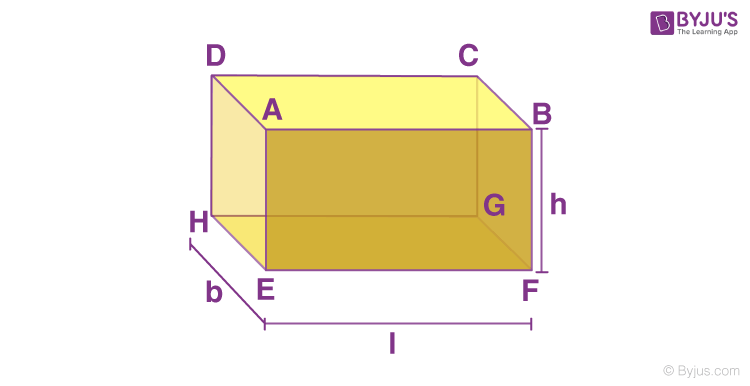The total surface area of the cuboid (TSA) = Sum of the areas of all its six faces

TSA (cuboid) = 2(× b2(× h2(× h2(lblh)

Lateral surface area (LSA) is the area of all the sides apart from the top and bottom faces.

The lateral surface area of the cuboid = Area of face AEHD + Area of face BFGC + Area of face ABFE + Area of face DHGC

LSA (cuboid) = 2(× h2(× h2h(b)

Length of diagonal of a cuboid =√(l+ b+ h2)

To know more about the Surface Area of Cuboid, visit here.

### Volume of a Cuboid

The volume of a cuboid is the space occupied within its six rectangular faces.

Volume of a cuboid (base area× heigh(lb)lbh

## Surface Area and Volume of Cube

A cube is a three-dimensional solid that has six square faces, twelve edges and eight vertices.

### Surface Area of Cube

As we know, one of the important properties of a cube is length = breadth = height.

If we assume that the length of the cube is “l”, and hence we get

So, obviously, here we get,

Height = l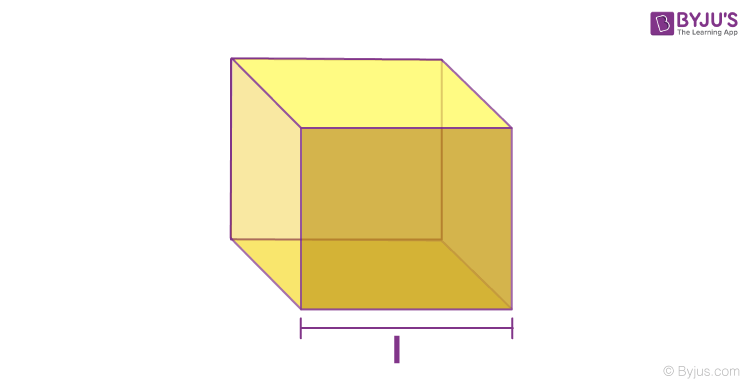The total surface area of the cube (TSA) = Sum of the areas of all its six faces.

In case of all faces has an equal area, TSA  of Cube = 6 × area of Square = 6l2 square units.

Similarly, the Lateral surface area of cube 2(× × l4l2
Note: Diagonal of a cube =√3l

### Volume of a Cube

Volume of a cube = base are× height
Since all dimensions of a cube are identical, volume = l3
Where l is the length of the edge of the cube.

To know more about the Volume of Cube and Cuboid, visit here.

#### For more information on Cube And Cuboid, watch the below videoTo know more about the Surface Area of Cube, visit here.

## Surface Area and Volume of Cylinder

A cylinder is a solid shape that has two circular bases connected with each other through a lateral surface. Thus, there are three faces, two circular and one lateral, of a cylinder. Based on these dimensions, we can find the surface area and volume of a cylinder.

### Surface Area of Cylinder

Take a cylinder of base radius r and height h units. The curved surface of this cylinder, if opened along the diameter (d = 2r) of the circular base can be transformed into a rectangle of length 2πr and height h units. Thus,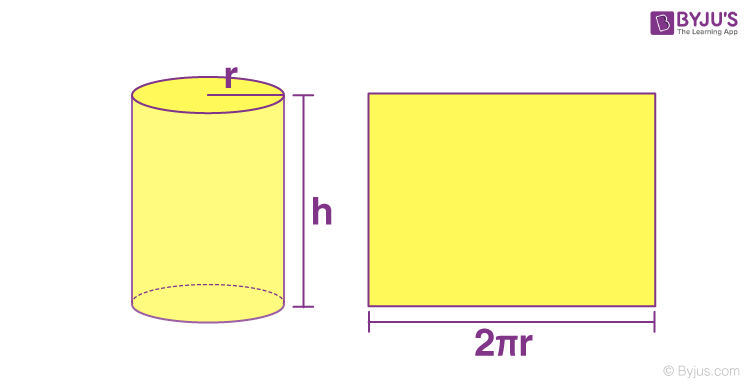CSA of a cylinder of base radius r and height 2π × × h
TSA  of a cylinder of base radius r and height 2π × × h + area of two circular bases
=2π × × 2πr2
=2πr(r)

### Volume of a Cylinder

Volume of a cylinder = Base area × height = (πr2× πr2h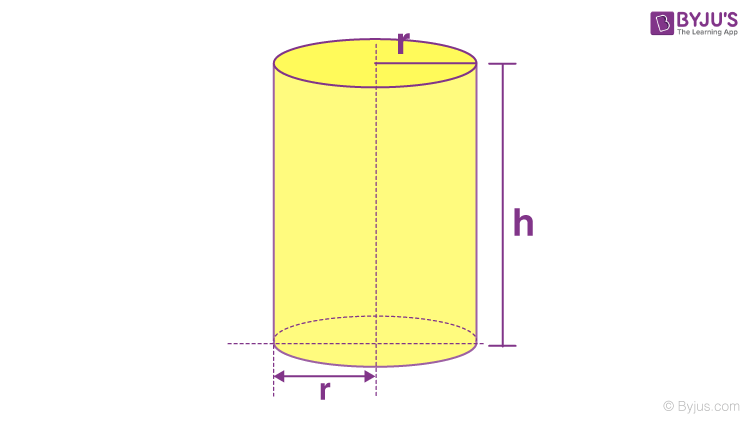To know more about the Volume of a Cylinder, visit here.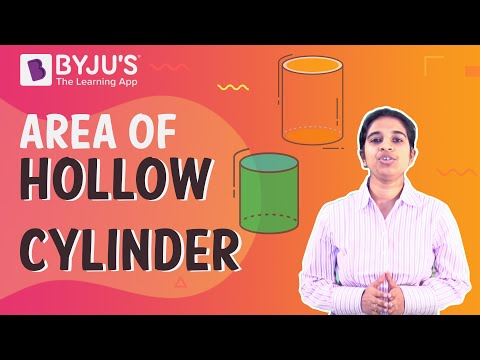To know more about the Surface Area of a Cylinder, visit here.

## Surface Area and Volume of Right Circular Cone

A cone is a 3d shape that has one circular base and narrows smoothly from the base to a point called the vertex.

### Surface Area of Cone

Consider a right circular cone with slant length l, radius r and height h.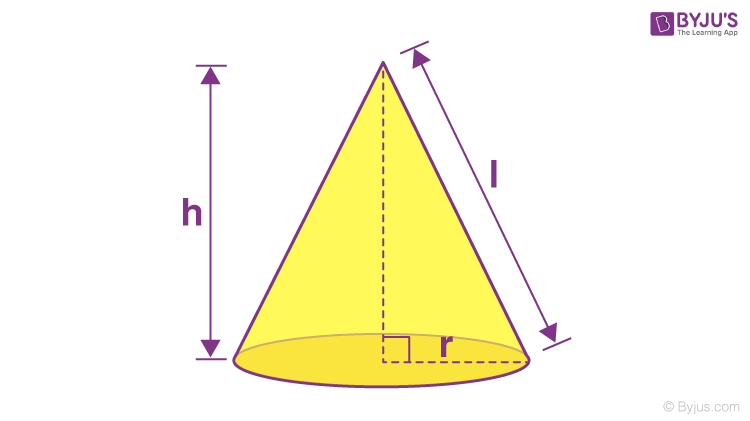CSA of right circular cone πrl
TSA = CSA + area of base πrπrπr(r)

To know more about the Surface Area of a Right Circular Cone, visit here.

### Volume of a Right Circular Cone

The volume of a Right circular cone is 1/3 times that of a cylinder of the same height and base.
In other words, 3 cones make a cylinder of the same height and base.
The volume of a Right circular cone =(1/3)πr2h
Where ‘r’ is the radius of the base and ‘h’ is the height of the cone.

To know more about the Volume of a Right Circular Cone, visit here.

## Surface Area and Volume of Sphere

A sphere is a solid that is round in shape, and the points on its surface are equidistant from the center.

### Surface Area of Sphere

For a sphere of radius r

Curved Surface Area (CSA) = Total Surface Area (TSA) = 4πr2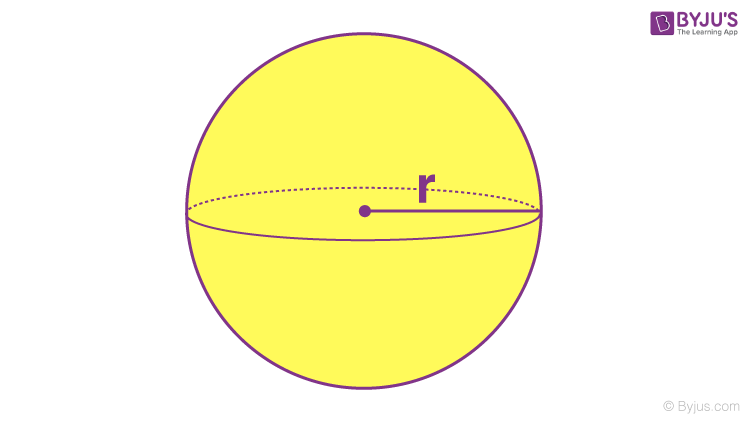#### For more information on Sphere And Hemisphere, watch the below video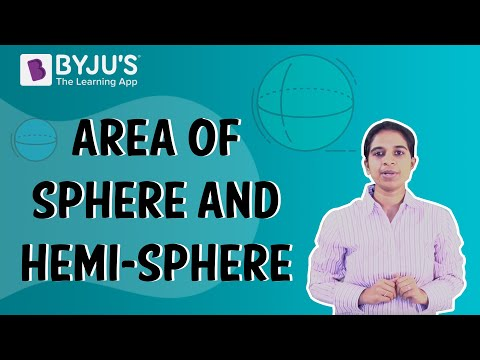### Volume of Sphere

The volume of a sphere of radius r = (4/3)πr3

To know more about volume of a Sphere, visit here.

## Surface Area and Volume of Hemisphere

A hemisphere is a shape that is half of the sphere and has one flat surface. The other side of the hemisphere is shaped as a circular bowl. See the figure below.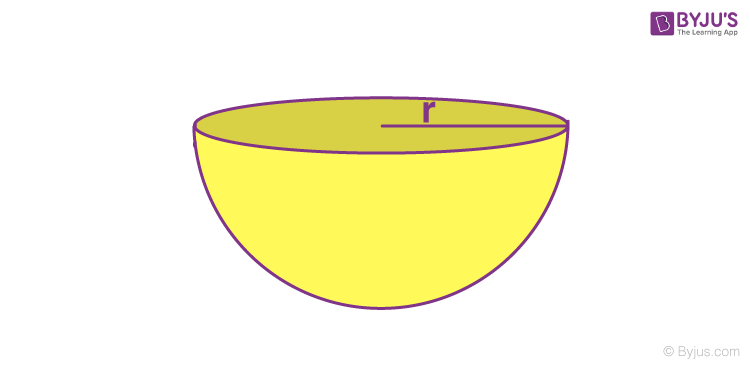### Surface Area of Hemisphere

We know that the CSA of a sphere  4πr2.

A hemisphere is half of a sphere.
CSA of a hemisphere of radius r 2πr2
Total Surface Area = curved surface area + area of the base circle
TSA 3πr2

To know more about Surface Area of Hemisphere, visit here.

### Volume of Hemisphere

The volume (V) of a hemisphere will be half of that of a sphere.
The volume of the hemisphere of radius r (2/3)πr3

To know more about Volume of Hemisphere, visit here.

## Surface Area and Volume of Combination of Solids

The combination of solids explains the shapes formed when two different solids are combined together. Thus, the surface area and volume for such shapes will vary from the other basic solids.

#### For more information on the Combination of Solids, watch the below video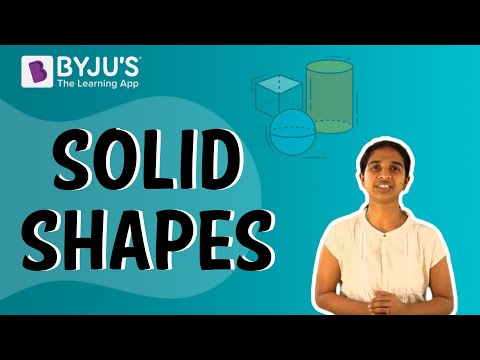### Surface Area of Combined Figures

Areas of complex figures can be broken down and analysed as simpler known shapes. By finding the areas of these known shapes, we can find out the required area of the unknown figure.
Example: 2 cubes each of volume 64 cm3  are joined end to end. Find the surface area of the resulting cuboid.
Length of each cube 64(1/3) 4cm
Since these cubes are joined adjacently,  they form a cuboid whose length l = 8 cm. But height and breadth will remain the same = 4 cm.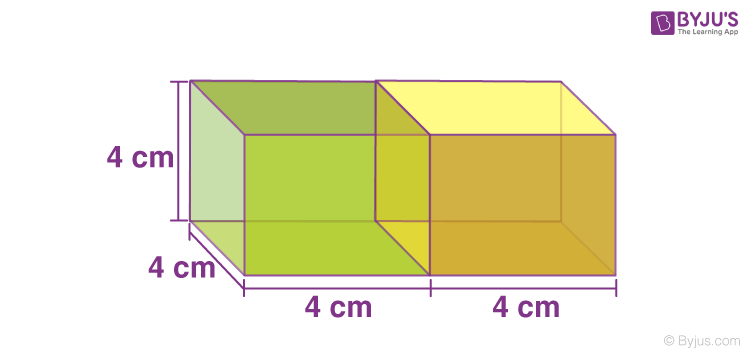The new surface area, TSA = 2(lb + bh + lh)

TSA = 2 (8 x 4 + 4 x 4 + 8 x 4)

= 2(32 + 16 + 32)

= 2 (80)

TSA = 160 cm2

### Volume of Combined Solids

The volume of complex objects can be simplified by visualising them as a combination of shapes of known solids.
Example: A solid is in the shape of a cone standing on a hemisphere with both their radii being equal to 3 cm and the height of the cone is equal to 5 cm.
This can be visualised as follows :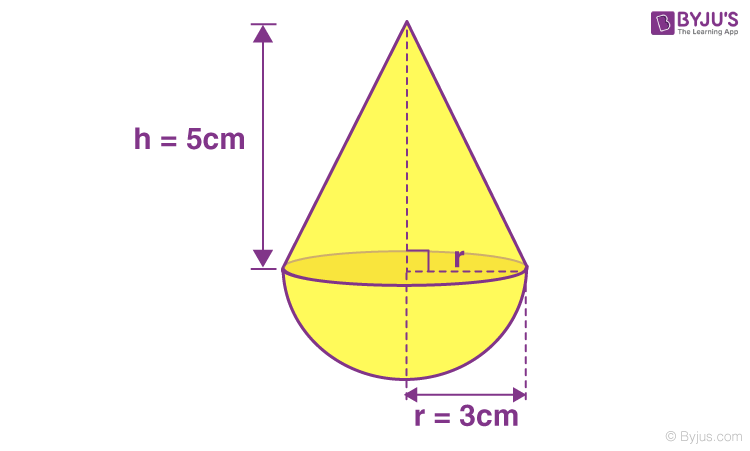V(solid) = V(Cone) + V(hemisphere)

V(solid) = (1/3)πr2h + (2/3)πr3

V(solid) = (1/3)π(9)(5) + (2/3)π(27)

V(solid) = 33π cm3

To know more about the Volume of a Combination of Solids, visit here.

## Video Lesson on Combination of Solids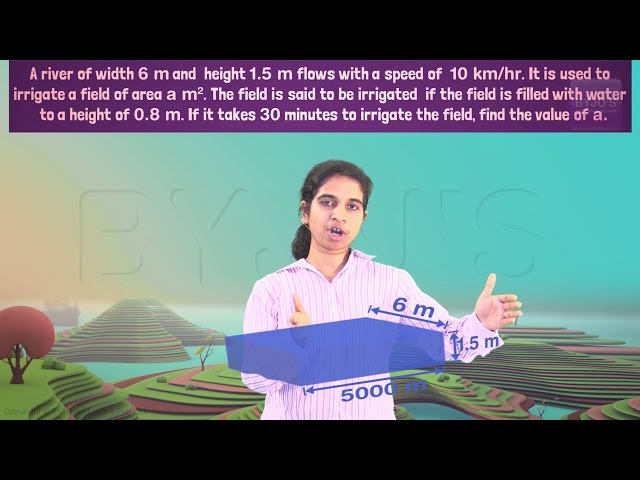## Surface Area and Volume of Frustum of a cone

When a solid is cut by a plane, then another form of solid is formed. One such form of a solid is the frustum of a cone, which is formed when a plane cuts a cone parallelly to the base of the cone. Let us discuss its surface area and volume here.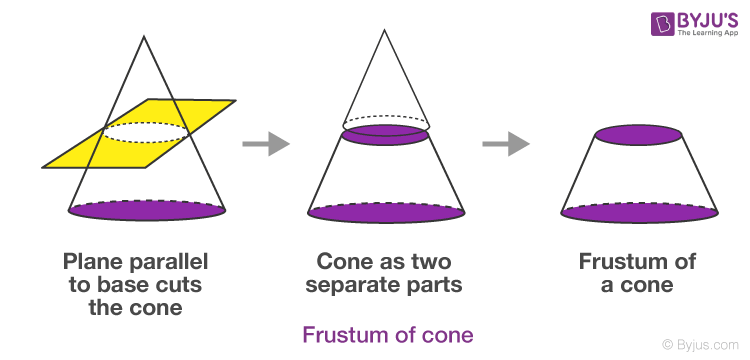If a right circular cone is sliced by a plane parallel to its base, then the part with the two circular bases is called a Frustum.

### Surface Area of a Frustum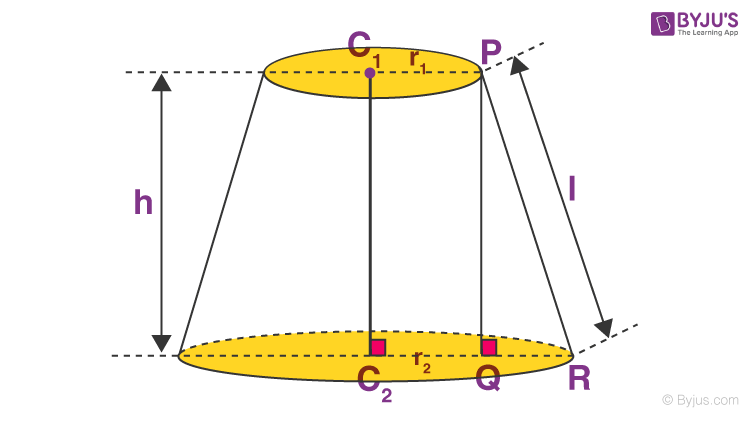CSA of frustum =π(r1+r2)l,  where l= √[h2+(r– r1)2]

TSA of the frustum is the CSA + the areas of the two circular faces = π(rr2)π(r12 r22)

Example:

Find the curved surface area of the frustum if the slant height of the cone’s frustum is 4 cm. Also, given that the circumferences of its circular ends are 6 cm and 18 cm.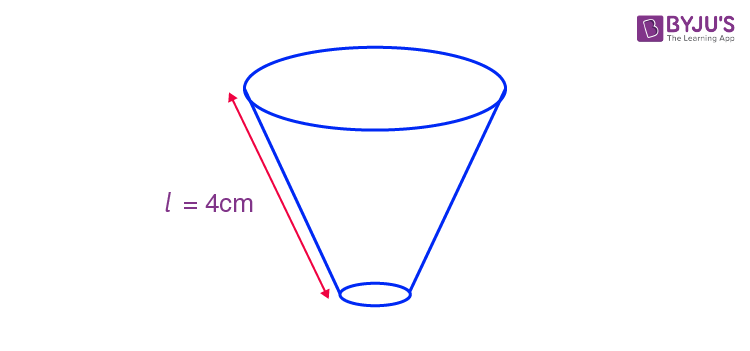Solution:

Given: Slant height, l = 4 cm

The circumferences of the two circular ends are 6 cm and 18 cm.

Let the radius of the two circular ends be r1 and r2.

Now, we have to find r1 and r2.

Finding r1:

Circumference of one end = 6 cm

2πr1 = 6

r1 = 6/2π

r1 = 3/π

Finding r2:

Circumference of the other end = 18 cm

2πr2 = 18

r2 = 18/2π

r2 = 9/π

We know that the formula to find the curved surface area of frustum is π(r1 + r2)l square units.

Thus by substituting the known values, we get

CSA of frustum = π[(3/π) + (9/π)]4

CSA of frustum = 4π(12/π)

CSA of frustum = 48 cm2.

Therefore, the curved surface area of the frustum is 48 cm2.

### Volume of a Frustum

The volume of a frustum of a cone =(1/3)πh(r12 r2r1r2)

Example:

A glass is in the shape of the frustum of a cone with a height of 14 cm. The diameters of its two circular ends are 2 cm and 4 cm. Find the volume of the glass.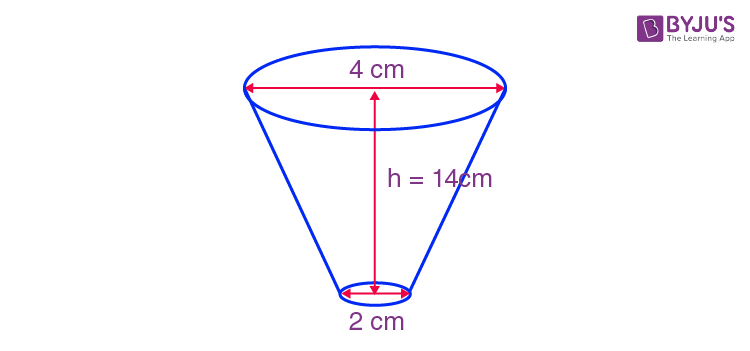Solution:

Given: Height of the frustum = 14 cm

The diameters of the two circular ends are 2 cm and 4 cm.

So, the radius will be 1 cm and 2 cm.

Let r1 = 1 cm and r2 = 2 cm

Since the glass is in the shape of a frustum, the volume of a glass = volume of a frustum.

Therefore, the volume of glass = (⅓)πh (r12 + r22 + r1r2) cubic units.

Now, substitute the values in the formula, we get

Volume of a glass, V = (⅓) π(14)[12 + 22 + (1)(2)]

V = (⅓) (22/7)(14) [1 + 4 + 2]

V = (⅓) (22/7)(14)(7)

V = [22 × 14 × 7] / [3 × 7]

V = (22 × 14 ) / 3

V = 308 / 3

V = 102. 67

Hence, the volume of a glass is 102. 67 cm3.

To know more about Frustum, visit here.

## Conversion of Solid from One Shape to Another

When a solid is converted into another solid of a different shape (by melting or casting), the volume remains constant.

Example:

A metallic sphere with a radius of 4.2 cm is melted and recast in the shape of a cylinder with a radius of 6 cm. Determine the cylinder’s height.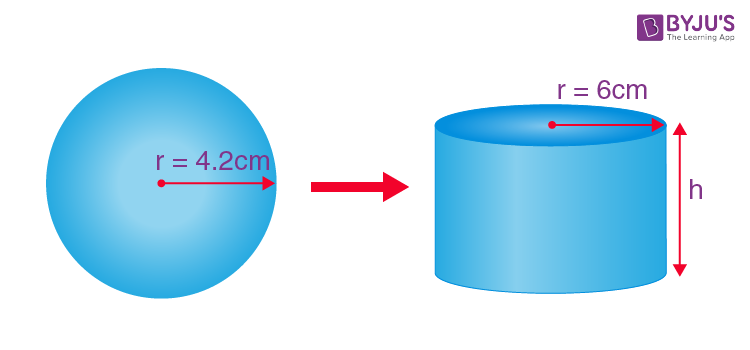Solution:

Given that, the sphere is melted and recast into a cylinder.

So, we can write,

Sphere’s volume = Cylinder’s volume.

As we know, the volume of a sphere = (4/3)πr3 cubic units.

And the volume of a cylinder = πr2h cubic units.

So, we can write:

(4/3)πr3 =  πr2h …(1)

Given that, the radius of the metallic sphere = 4.2 cm.

Radius of the cylinder = 6 cm.

Now, substitute the values in (1), we get

(4/3)π(4.2)3 =  π(6)2

(4/3)(4.2)3 = 36h

Hence, h = [(4/3)(4.2)3]/36

h = [4 × 4.2 × 4.2 × 4.2 ]/[3 × 36]

By simplifying the above expression, we get

h = 2.744 cm

Hence, the height of the cylinder is 2.744 cm.

To know more about the Shape Conversion of Solids, visit here

## Surface Areas and Volumes Formulas – Summary

The following table presents a brief summary of different solid shapes with their surface area and volume formulas:

 Shape Parameters Surface Area (Square units) Volume (Cubic units) Cuboid Length = l Breadth = b Height = h TSA = 2(lb + bh + lh) LSA = 2h(l + b) V = l × b × h Cube Length = Breadth = Height = l TSA = 6l2 LSA = 4l2 V = l3 Cylinder Radius = r Height = h CSA = 2π × r × h TSA = 2πr(h + r) V = πr2h Cone Radius = r Height = h Slant Height = l CSA = πrl TSA = πr(l + r) V = (1/3)πr2h Sphere Radius = r CSA = TSA =  4πr2 V = (4/3)πr3 Hemisphere Radius = r CSA = 2πr2 TSA = 3πr2 V =  (2/3)πr3 Frustum Radius of top circular part = r1 Radius of bottom circular part = r2 Height = h Slant height = l CSA = π(r1+r2)l TSA = π(r1 + r2)l + π(r12 + r22) V = (1/3)πh(r12 + r22 + r1r2)

Stay tuned to BYJU’S – The Learning App and download the app today to get all class-wise concepts.

Test your knowledge on Surface Area Volumes Class 10